Chapter 4 Class 10 Quadratic Equations

Class 10
Important Questions for Exam - Class 10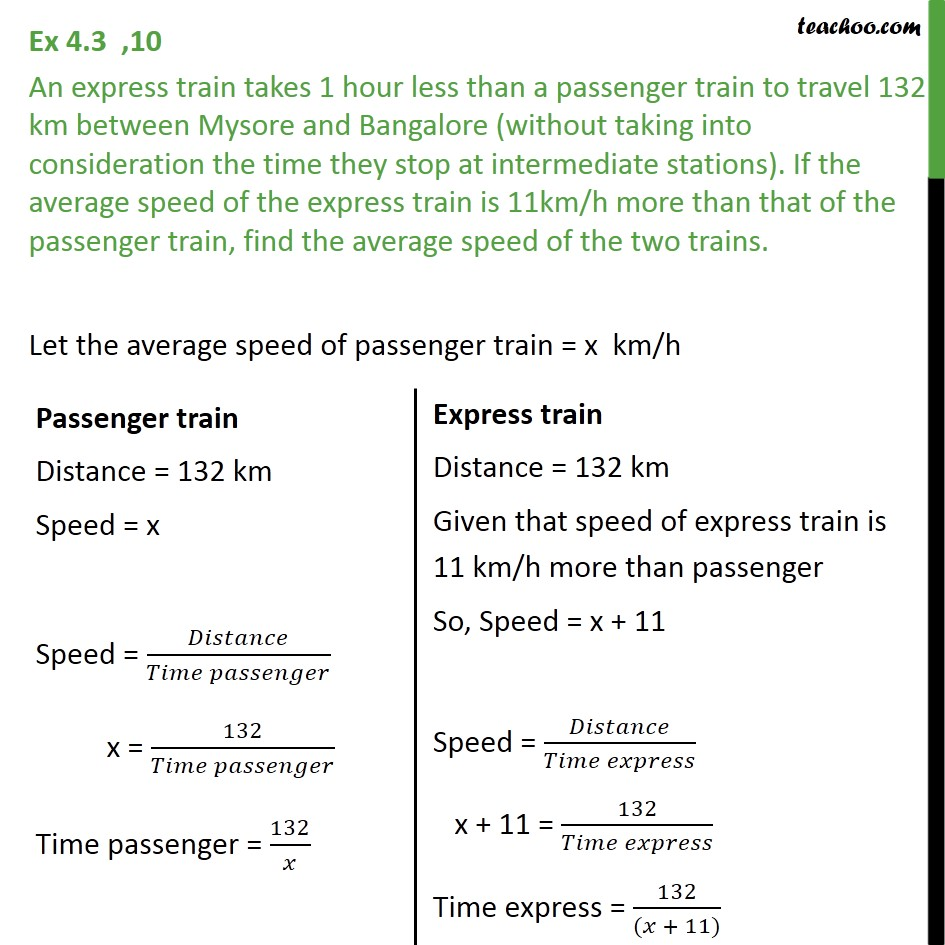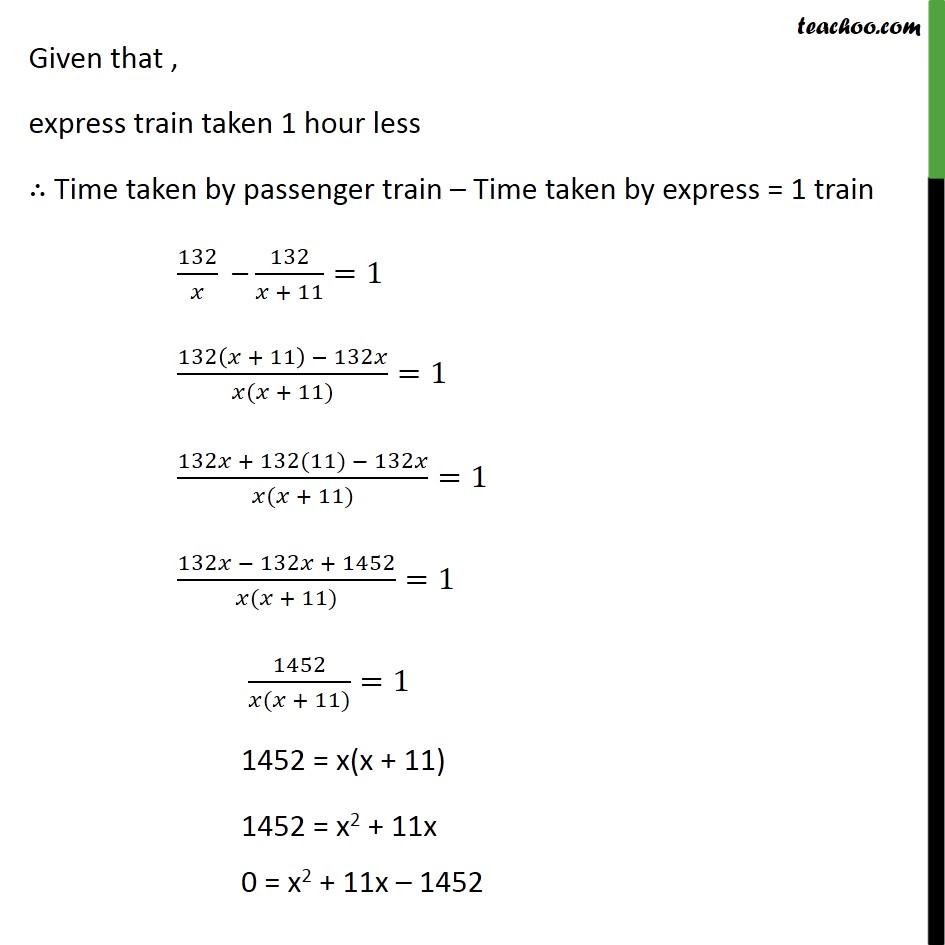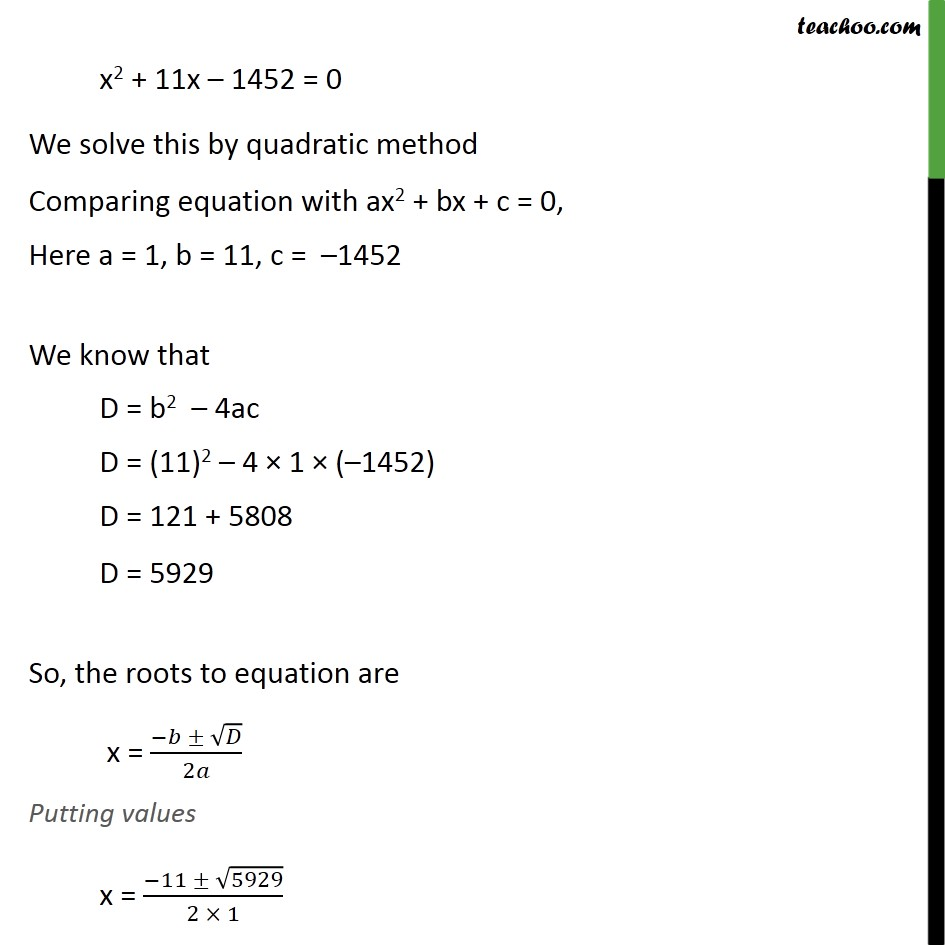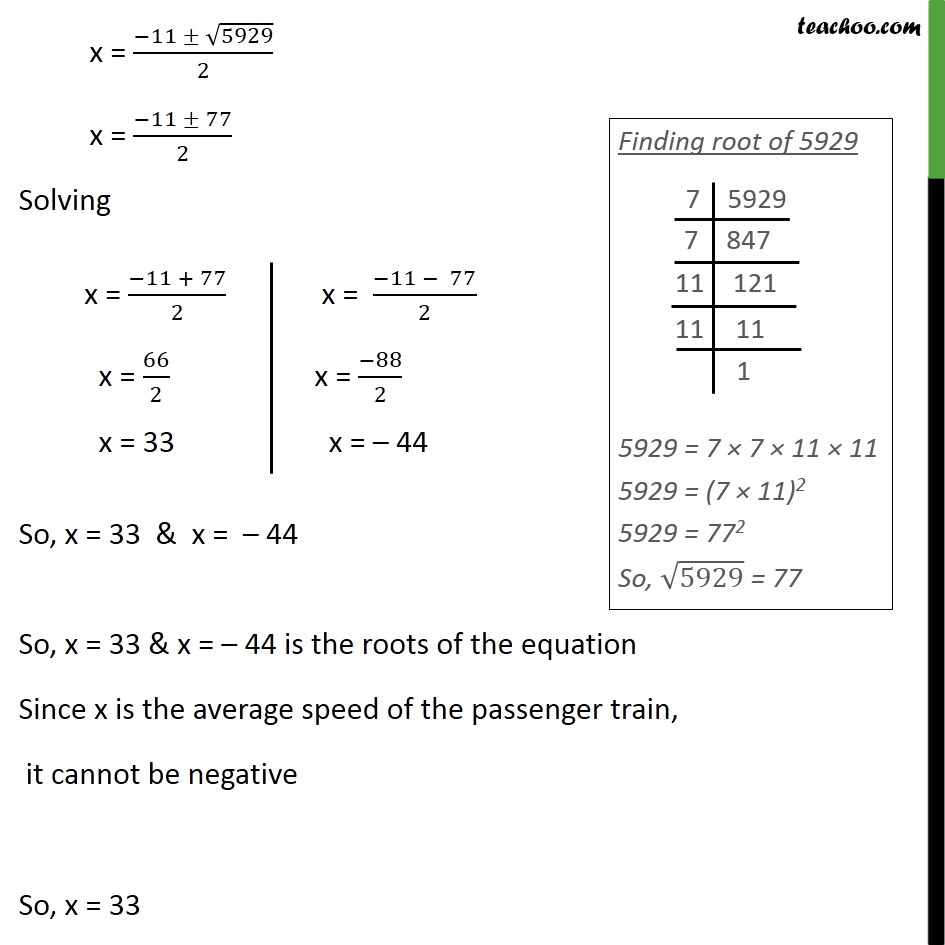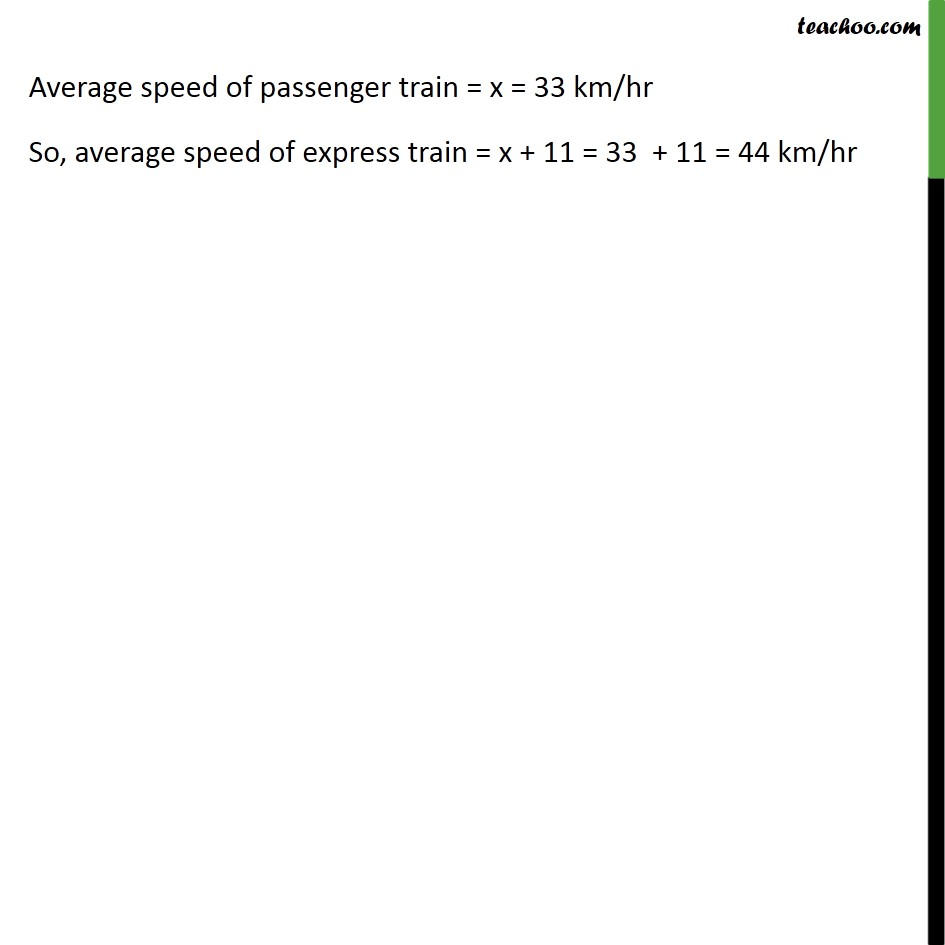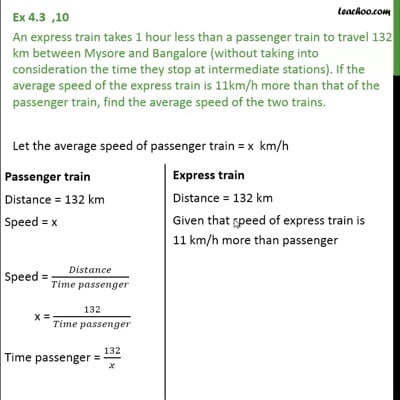This video is only available for Teachoo black users

Introducing your new favourite teacher - Teachoo Black, at only ₹83 per month

### Transcript

Ex 4.3 ,10 An express train takes 1 hour less than a passenger train to travel 132 km between Mysore and Bangalore (without taking into consideration the time they stop at intermediate stations). If the average speed of the express train is 11km/h more than that of the passenger train, find the average speed of the two trains. Let the average speed of passenger train = x km/h Given that , express train taken 1 hour less ∴ Time taken by passenger train – Time taken by express = 1 train 132/𝑥 – 132/(𝑥 + 11)=1 (132(𝑥 + 11) − 132𝑥)/(𝑥(𝑥 + 11))=1 (132𝑥 + 132(11) − 132𝑥)/(𝑥(𝑥 + 11))=1 (132𝑥 − 132𝑥 + 1452)/(𝑥(𝑥 + 11))=1 1452/(𝑥(𝑥 + 11))=1 1452 = x(x + 11) 1452 = x2 + 11x 0 = x2 + 11x – 1452 x2 + 11x – 1452 = 0 We solve this by quadratic method Comparing equation with ax2 + bx + c = 0, Here a = 1, b = 11, c = –1452 We know that D = b2 – 4ac D = (11)2 – 4 × 1 × (–1452) D = 121 + 5808 D = 5929 So, the roots to equation are x = (−𝑏 ± √𝐷)/2𝑎 Putting values x = (−11 ± √5929)/(2 × 1) x = (−11 ± √5929)/2 x = (−11 ± 77)/2 Solving So, x = 33 & x = – 44 So, x = 33 & x = – 44 is the roots of the equation Since x is the average speed of the passenger train, it cannot be negative So, x = 33 Average speed of passenger train = x = 33 km/hr So, average speed of express train = x + 11 = 33 + 11 = 44 km/hr